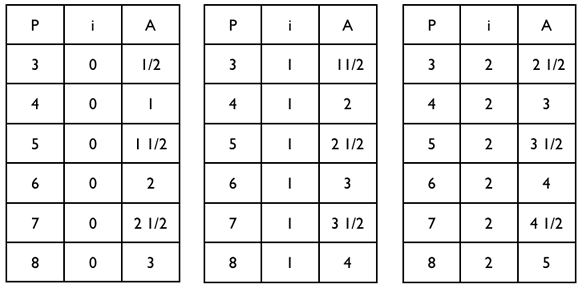#### You may also like### Consecutive Numbers

An investigation involving adding and subtracting sets of consecutive numbers. Lots to find out, lots to explore.### Calendar Capers

Choose any three by three square of dates on a calendar page...### Days and Dates

Investigate how you can work out what day of the week your birthday will be on next year, and the year after...

# Pick's Theorem Poster

##### Age 11 to 14Challenge Level

The formula linking $p, i$ and $A$ is $A = \frac12p + i - 1$

Trying out different shapes, you can find a pattern:$p$ increase by $1$ $\Rightarrow A$ increase by $\frac12$ (down table)
$i$ increase by $1$ $\Rightarrow A$ increase by $1$ (across table)

So $A = \frac12p + i - 1$